# 2. Thermal Effects in Semiconductor Devices

Alles Vergängliche ist nur ein Gleichnis.''

Johann Wolfgang von Goethe2.1

MANY challenges faced in modern semiconductor devices are related to heating phenomena. Since shrinking the device feature size causes higher power loss densities and therefore higher and faster temperature evolution inside the device structure, many additional problems occur due to material-related constraints because the produced heat cannot be transported to the heat sink fast enough. Hence the surrounding device structure heats up and the global microelectronic chip heats up globally.

Since the absolute temperature is not zero, matter is in steady motion at least in terms of BROWN's2.2 molecular movements. Therefore, the most probable consequence is that the number of possible states of a closed system, e.g the quantum states, is increasing until a temporary state of thermal equilibrium has been reached. Hence, also the entropy which represents the information about the reachable states in a system is not decreasing spontaneously. This fundamental theorem of thermodynamics and its derivations challenge today's electronic devices including the decrease of the device feature size on the wafer while the ITRS request that the operational current density remains the same. Correspondingly, the power loss density increases quadratically with the reduction of the feature size. Two possible alternatives to overcome these problems are to reduce the supply voltage or the use of alternative materials which produce and inherit less parasitic effects. To describe the general behavior of the electro-magnetic system, fundamental electro-magnetic field equations are given by MAXWELL2.3 [58,59,60] as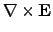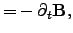(2.1)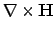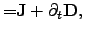(2.2)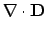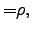(2.3)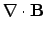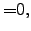(2.4)

which are independent of the temperature and independent of the type of matter. The quantities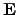and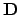are the electric field and the displacement vector whileand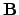are the magnetic field and the magnetic flux density vector, respectively.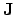represents the current density and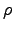the electric space charge density. Information about the material properties is included via the material equations for each region of matter by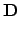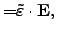(2.5)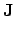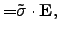(2.6)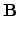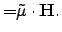(2.7)

With these equations, the field quantities and the flux quantities are coupled via the material-dependent parameters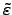,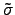, and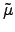. Here,denotes the permittivity tensor,the conductivity tensor, andthe permeability tensor. While the MAXWELL equations describe only field properties and field physics, the introduced material parameter tensors,, andreflect the material physics and describe the observed material-related phenomena within the involved materials. To complete the equations for the material parameters the heat conduction equation can be derived from FOURIER's2.4 law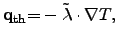(2.8)

in which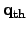represents the local heat flux density. This quantity is proportional to the temperature gradient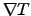, where the proportionality factor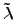is the thermal conductivity tensor. In conjunction with (2.8), the energy conservation law can be expressed by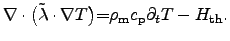(2.9)

Here,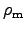denotes the mass density,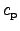the specific heat,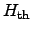is the heat generation term, and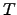the local temperature.

The heat conduction equation (2.9) has some critical quantities: the heat generationterm, the thermal conductivity, and the specific heat capacitance. The heat generation termis mostly represented by JOULE's2.5 power loss (cf. Section 2.2.3). For the thermal conductivityand the specific heat capacitanceseveral tables of material parameters exist, which have also different ranges of validity (cf. Section 2.2.1).

In order to describe the behavior of semiconductor devices more specifically, the necessary equations can be derived from MAXWELL's equations . Equation (2.3) and (2.2) are used to derive the continuity equation between the charge carrier current for space charge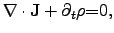(2.10)

where the space charge densitycan be described by the different contributing charge carriers and the net doping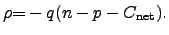(2.11)

Here,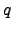denotes the elementary charge and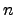,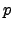, and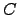are the carrier densities for electrons and holes and the net doping density, respectively. The net doping itself can be split into acceptor and donor doping concentrations to account for different dopant species. With a carrier generation rate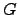, equation (2.10) can be finally separated into equations for current densities for electrons and holes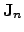and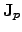, respectively: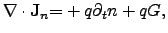(2.12)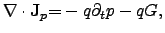(2.13)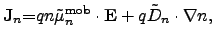(2.14)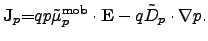(2.15)

In these equations,anddenote the carrier concentration for electrons and holes,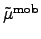is the carrier mobility tensor,the electric field vector, and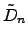and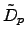are the diffusion coefficient tensors for electrons and holes, respectively. The equation set (2.12)-(2.15) denotes the drift diffusion model as a solution of the BOLTZMANN2.6 transport equation using the first two moments of the distribution function, a parabolic dispersion relation, and the macroscopic relaxation time approximation [63,62].

By introducing an electrical potential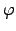as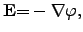(2.16)

where the electric fieldis expressed as the spatial gradient of the electrostatic potential, (2.3) reads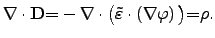(2.17)

For homogeneous materials, the material tensorcan be approximated by a scalar-valued quantity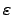, equation (2.17) degenerates to the well known POISSON 2.7 equation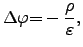(2.18)

where the electrical potential is determined by the space charge concentration and the dielectric constant in the matter within the specified simulation domain.

More problem-specific models have to be introduced instead of the generally used ones to described the discrepancies between reality and the observed model behavior. For instance, if the transient behavior of a clock frequency shift of an oscillator has to be considered during different operation conditions, many additional thermal and transient phenomena occur and influence the device behavior significantly . Therefore, the applied simulation models have to be adapted for each particular case appropriately to achieve an accurate problem description.

The following part of this chapter gives an overview of the most important parts of the thermodynamics in semiconductor devices with respect of their application to industrial-relevant examples [65,66,62,67,68,69].

Subsections
Stefan Holzer 2007-11-19